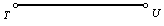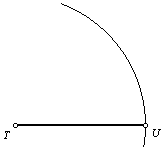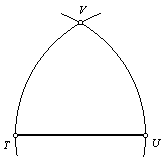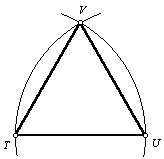Given a line segment as one side, construct an equilateral triangle.

This method may also be used to construct a 60° angle.

 1. Begin with line segment TU.2. Center the compass at point T, and set the compass radius to TU. Draw an arc as shown.3. Keeping the same radius, center the compass at point U and draw another arc intersecting the first one. Let point V be the point of intersection.4. Draw line segments TV and UV. Triangle TUV is an equilateral triangle, and each of its interior angles has a measure of 60°.Back to Geometry Construction Reference